# SURVEY OF CHEMISTRY LABORATORY I CHEM 1151 L

• Slides: 15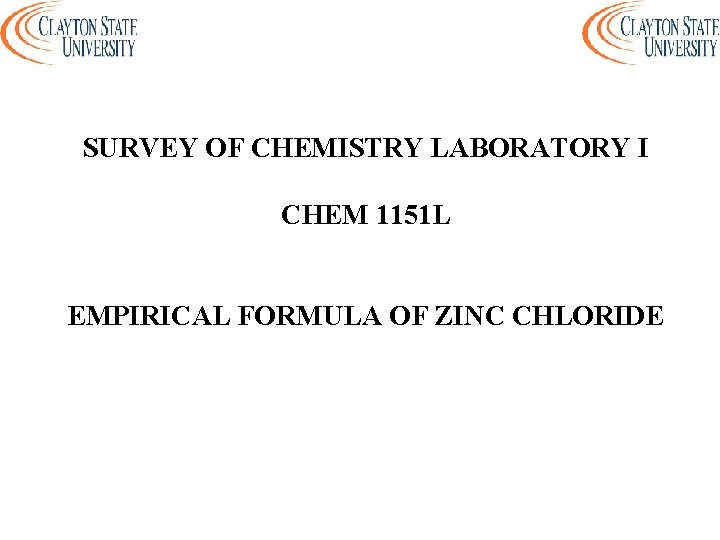SURVEY OF CHEMISTRY LABORATORY I CHEM 1151 L EMPIRICAL FORMULA OF ZINC CHLORIDE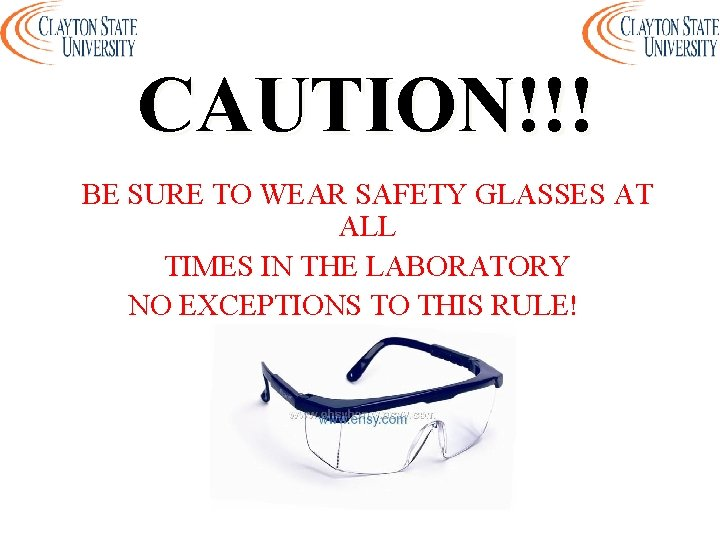CAUTION!!! BE SURE TO WEAR SAFETY GLASSES AT ALL TIMES IN THE LABORATORY NO EXCEPTIONS TO THIS RULE!EMPIRICAL FORMULA OF ZINC CHLORIDE Students will determine - the empirical formula of zinc chloride by a process known as synthesis - the empirical formula from their data - the empirical formula from the class average It is best to perform several experiments and obtain average values for the result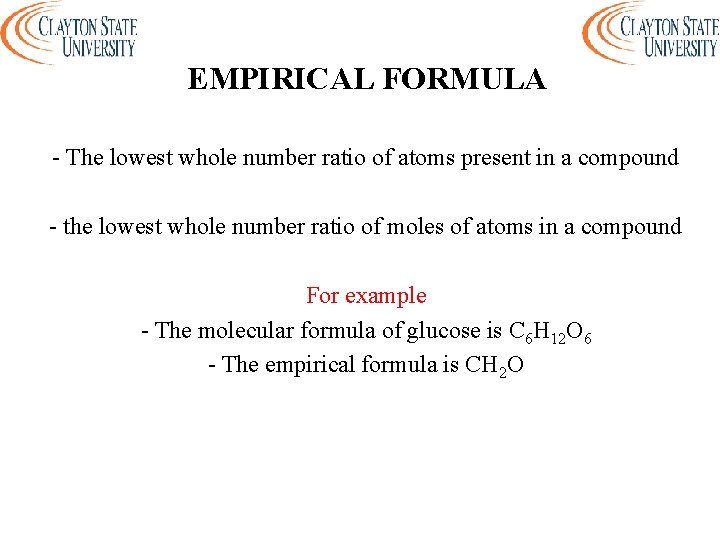EMPIRICAL FORMULA - The lowest whole number ratio of atoms present in a compound - the lowest whole number ratio of moles of atoms in a compound For example - The molecular formula of glucose is C 6 H 12 O 6 - The empirical formula is CH 2 O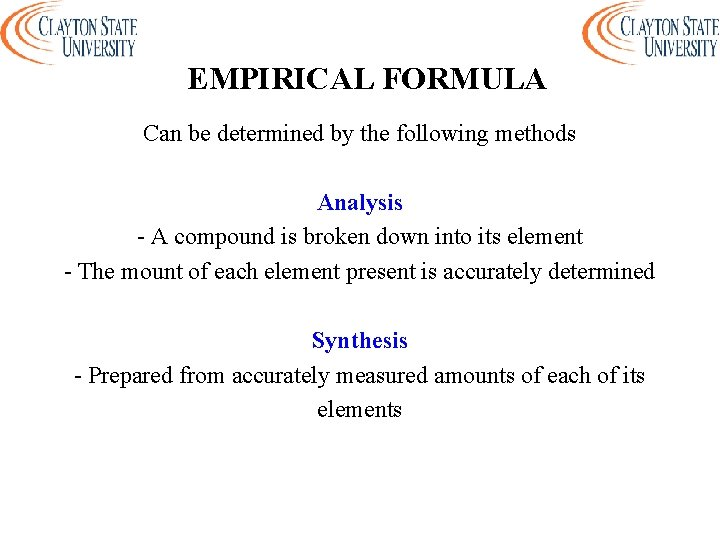EMPIRICAL FORMULA Can be determined by the following methods Analysis - A compound is broken down into its element - The mount of each element present is accurately determined Synthesis - Prepared from accurately measured amounts of each of its elementsEMPIRICAL FORMULA - Determine the mass of each element found in the formula (may be grams or percentage composition by mass) - Convert each mass to moles using the atomic masses of the appropriate elements - Divide each mole amount by the smallest number of moles - Round each mole ratio to the nearest integer - Write the whole numbers as subscripts in the formula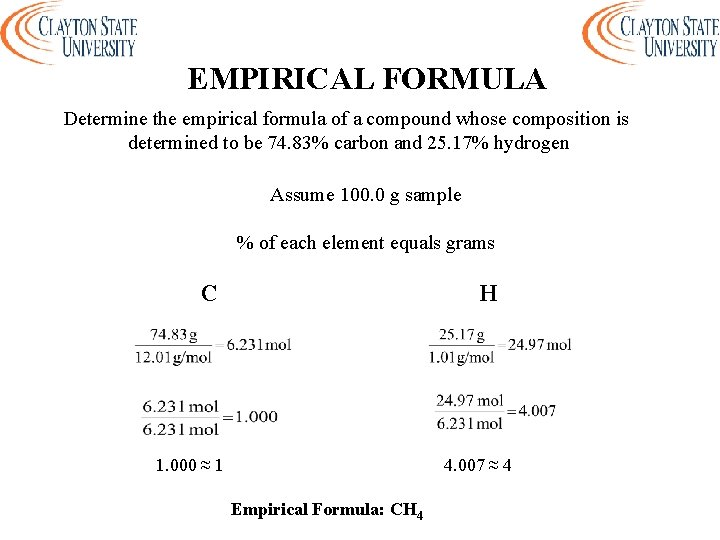EMPIRICAL FORMULA Determine the empirical formula of a compound whose composition is determined to be 74. 83% carbon and 25. 17% hydrogen Assume 100. 0 g sample % of each element equals grams C H 1. 000 ≈ 1 4. 007 ≈ 4 Empirical Formula: CH 4ZINC METAL - Mass about 1 gram of zinc metal - Record this massEVAPORATING DISH - Mass a clean, dry evaporating dish - Record this mass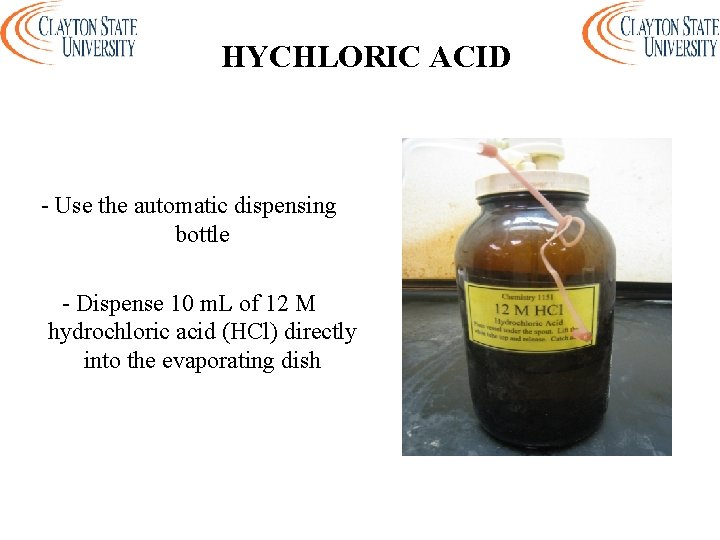HYCHLORIC ACID - Use the automatic dispensing bottle - Dispense 10 m. L of 12 M hydrochloric acid (HCl) directly into the evaporating dishHYCHLORIC ACID + ZINC METAL - Carefully add the zinc to the dish containing the HCl - Must be done in the hood - Allow all of the zinc to dissolve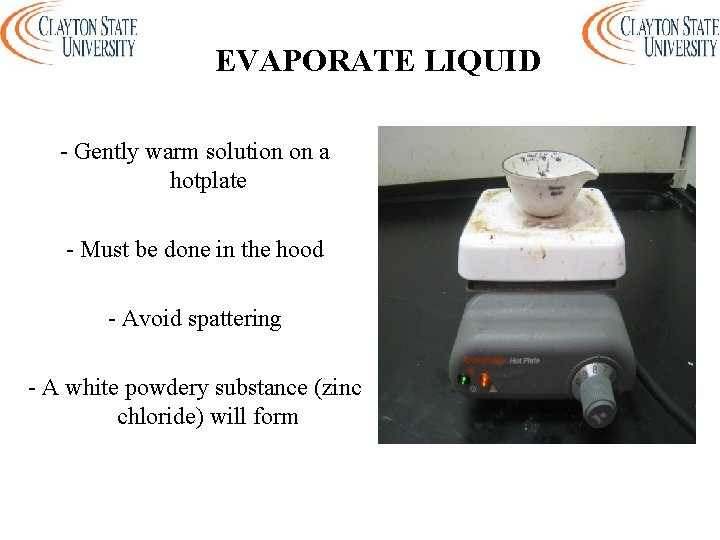EVAPORATE LIQUID - Gently warm solution on a hotplate - Must be done in the hood - Avoid spattering - A white powdery substance (zinc chloride) will form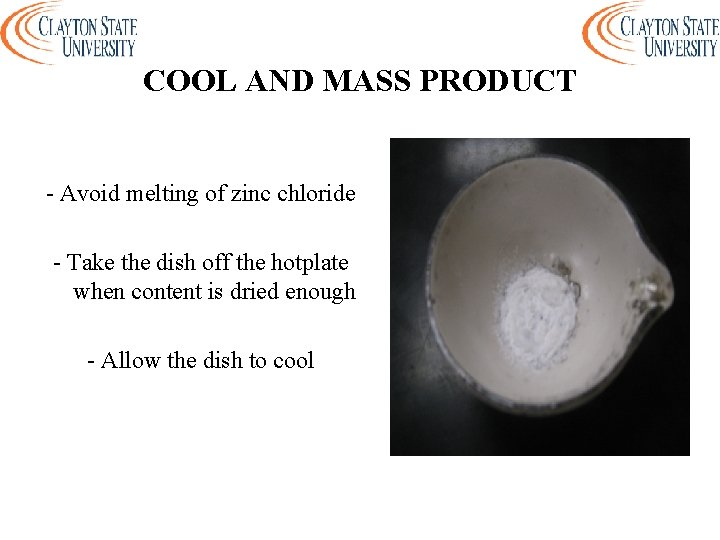COOL AND MASS PRODUCT - Avoid melting of zinc chloride - Take the dish off the hotplate when content is dried enough - Allow the dish to coolCOOL AND MASS PRODUCT - Mass the dish and the zinc chloride - Record the mass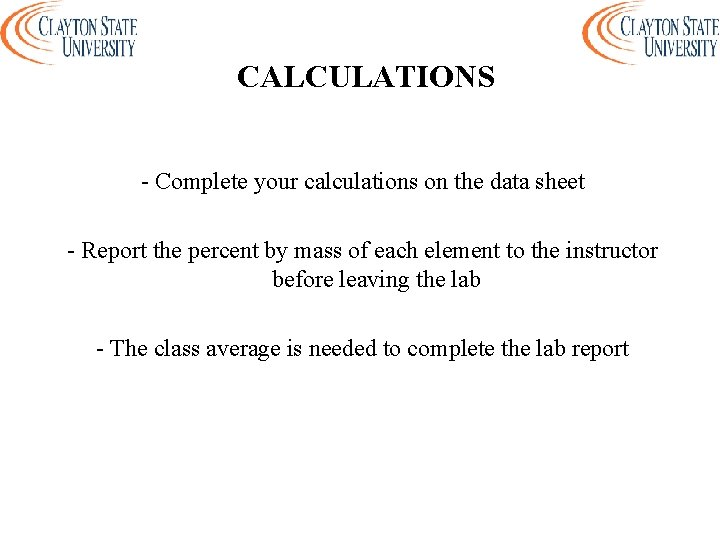CALCULATIONS - Complete your calculations on the data sheet - Report the percent by mass of each element to the instructor before leaving the lab - The class average is needed to complete the lab report